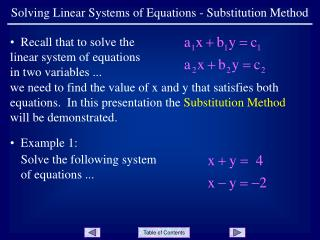DownloadDownload PresentationSolving Linear Systems of Equations - Substitution Method

# Solving Linear Systems of Equations - Substitution Method

Download Presentation## Solving Linear Systems of Equations - Substitution Method

- - - - - - - - - - - - - - - - - - - - - - - - - - - E N D - - - - - - - - - - - - - - - - - - - - - - - - - - -
##### Presentation Transcript

1. Solving Linear Systems of Equations - Substitution Method • Recall that to solve the linear system of equations in two variables ... we need to find the value of x and y that satisfies both equations. In this presentation the Substitution Method will be demonstrated. • Example 1: Solve the following system of equations ...

2. Solving Linear Systems of Equations - Substitution Method • Label the equations as • # 1 and # 2. • Start with equation # 2 and solve for x ... • Since y - 2 is the same value as x, we can substitute y - 2 for x in equation # 1 ... Slide 2

3. Solving Linear Systems of Equations - Substitution Method • Solve this equation for y ... • Substitute this value for y in equation # 2 and solve for x ... • The solution to the system is (1,3), or x = 1 y = 3 Slide 3

4. Solving Linear Systems of Equations - Substitution Method • Summary: 1) Solve for one variable in either equation. Choose a variable that has a coefficient of 1 if possible. 2) Substitute the expression for the variable in the other equation. This leaves one equation in one unkown, for which we can solve. 3) Substitute the given value for the variable into either equation and solve for the other variable. 4) To check the solutions, substitute both values into the equation that was not used in step 3. Slide 4

5. Solving Linear Systems of Equations - Substitution Method • Example 2: Solve the following system of equations ... • Solve the second equation for y ... Slide 5

6. Solving Linear Systems of Equations - Substitution Method • Substitute the expression for y in equation # 1 ... and solve for x ... Slide 6

7. Solving Linear Systems of Equations - Substitution Method • Substitute the value for x into equation # 1 (either equation could be used at this point) ... and solve for y ... • The solution to the system is ... Slide 7

8. Solving Linear Systems of Equations - Substitution Method • Since equation # 1 was used in the last step, check by substituting the values into equation # 2 ... Slide 8

9. Solving Linear Systems of Equations - Substitution Method END OF PRESENTATION Click to rerun the slideshow.